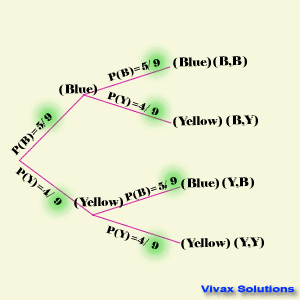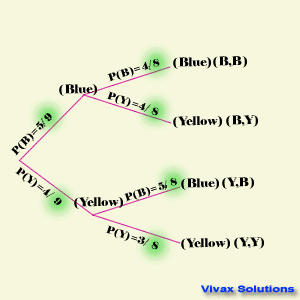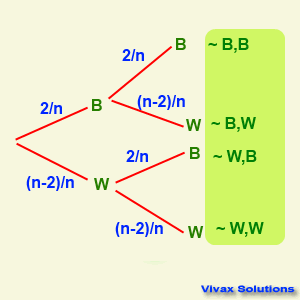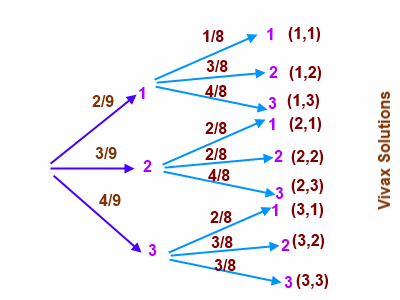# Mathematics

### Tree Diagrams

Tree diagrams show a clear pattern of probabilities in branches rather than in a table; the product of the probabilities of along a branch gives the probability of the event of that branch. The following examples show how the way they are used in two different situations.

E.g.1

There are 5 blue balls and 4 yellow balls in a container. A ball is taken out at random, colour noted and put back in. A second ball is taken out, and the procedure is repeated. Calculate the probability of getting two balls of different colours.P(B,Y) = 5/9 X 4/9 = 20 / 81
P(Y,B) = 4/9 X 5/9 = 20 /81
Since events (B,Y) and (Y,B) cannot happen at the same time, they are mutually exclusive. Therefore, 'OR' rule can be applied.
P(Balls of different colours) = 20/81 + 20/81 = 40/81

E.g.2

There are 5 blue balls and 4 yellow balls in a container. A ball is taken out at random, colour noted and not put back in. A second ball is taken out, and the procedure is repeated. Calculate the probability of getting two balls of different colours and two blue balls.On the second draw, the total number of balls has gone down by one and so has the number of the balls in the previous draw.
P(B,Y) = 5/9 X 4/8 = 20 /72
P(Y,B) = 4/9 X 5/8 = 20 /72
Since events (B,Y) and (Y,B) cannot happen at the same time, they are mutually exclusive. Therefore, 'OR' rule can be applied.
P(Balls of different colours) = 20/72 + 20/72 = 40/72 = 5/9
P(B,B) = 5/9 X 4/8 = 20 /72 = 5/9

E.g.3

There are n balls in a container; 2 of them are black and the rest are white. A ball is taken out at random, colour noted and put it back in. Then, a second ball is taken and the steps are repeated. The probability of getting two balls of different colours turned out to be 12/25. Show that 3n2 - 25n + 50 = 0. Hence, calculate the value of n.

The events can be shown by a tree diagram as follows:The probability of taking a ball of different color = 2/n X (n-2)/ n + 2/n X (n-2)/ n
= (4n - 8)/n2
(4n - 8)/n2 = 12/25
12n2 = 100n - 200
3n2 - 25n + 50 = 0
3n(n-5) - 10(n-5) = 0
(3n-10)(n-5) = 0
n = 5 or n = 10/3 = 3.3
Since, n has to be an integer, n = 5.

E.g.3

1
1
2
2
2
3
3
3
3

There are 9 plates with a certain number on each. Adam picks up a plate at random, marks the number and does not replace it, before picking up the second plate. By using a tree diagram, find the probability that
1) Both plates have number 2 on them
2) The number on the second plate is greater than the that on the first.1) (2,2) 3/9 X 2/8 = 6/72 = 1/12

2) P(1,2) OR P(1,3) OR P(2,3) = 2/9 X 3/8 + 2/9 X 4/8 + 3/9 X 4/8 = 6/72 + 8/72 + 12/72 = 26/72 = 13/36

Work out the following questions:

1. There are three red balls and five blue balls in a container. A ball is taken out at random, colour noted and put it back. A second ball is taken out at random, colour noted and the procedure is repeated. Find the probability of getting a ball of the same colour and a ball of different colour.
2. There are three red balls and five blue balls in a container. A ball is taken out at random, colour noted and not put back. A second ball is taken out at random, colour noted and the procedure is repeated. Find the probability of getting a ball of the same colour and a ball of different colour.
3. The probability of Sarah catching the bus is 0.6. The probability of her getting late to school is 0.3. Find the probability of catching the bus and being late to school.
4. The probability of getting a Head from a certain old coin is 2/3. It is tossed twice. Find the probability of getting a Head and Tail.
5. The letters of PEEL are in container. A letter is taken out, whether it is vowel or consonant is noted, and put back. This procedure is repeated once more. Find the probability of getting a vowel and consonant. Calculate the probability of getting two vowels as well.Maths is challenging; so is finding the right book. K A Stroud, in this book, cleverly managed to make all the major topics crystal clear with plenty of examples; popularity of the book speak for itself - 7th edition in print.

### Recommended - GCSE & iGCSEThis is the best book available for the new GCSE(9-1) specification and iGCSE: there are plenty of worked examples; a really good collection of problems for practising; every single topic is adequately covered; the topics are organized in a logical order.

### Recommended for A LevelThis is the best book that can be recommended for the new A Level - Edexcel board: it covers every single topic in detail;lots of worked examples; ample problems for practising; beautifully and clearly presented.